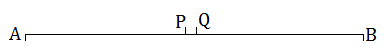Newbie

# In Question 4, point C is called a mid-point of line segment AB. Prove that every line segment has one and only one mid-point.Q.5

• 0

This is very important question of ncert class 9th of chapter Introduction To Euclid’s Geometry  . How I solve the best solution of exercise 5.1 question number 5. Please help me to solve this in a easy and best way.In Question 4, point C is called a mid-point of line segment AB. Prove that every line segment has one and only one mid-point.

Share

1. Solution:Let, AB be the line segment

Assume that points P and Q are the two different mid points of AB.

Now,

∴ P and Q are midpoints of AB.

Therefore,

AP = PB and AQ = QB.

also,

PB+AP = AB (as it coincides with line segment AB)

Similarly, QB+AQ = AB.

Now,

Adding AP to the L.H.S and R.H.S of the equation AP = PB

We get, AP+AP = PB+AP (If equals are added to equals, the wholes are equal.)

⇒ 2AP = AB — (i)

Similarly,

2 AQ = AB — (ii)

From (i) and (ii), Since R.H.S are same, we equate the L.H.S

2 AP = 2 AQ (Things which are equal to the same thing are equal to one another.)

⇒ AP = AQ (Things which are double of the same things are equal to one another.)

Thus, we conclude that P and Q are the same points.

This contradicts our assumption that P and Q are two different mid points of AB.

Thus, it is proved that every line segment has one and only one mid-point.

Hence Proved

• 0